GFG App
Open AppBrowser
Continue

# Working with Databases in R Programming

Prerequisite: Database Connectivity with R Programming

In R programming Language, a number of datasets are passed to the functions to visualize them using statistical computing. So, rather than creating datasets again and again in the console, we can pass those normalized datasets from relational databases.

## Databases in R Programming Language

R can be connected to many relational databases such as Oracle, MySQL, SQL Server, etc, and fetches the result as a data frame. Once the result set is fetched into data frame, it becomes very easy to visualize and manipulate them. In this article, we’ll discuss MySQl as reference to connect with R, creating, dropping, inserting, updating, and querying the table using R Language.

### RMySQL Package

It is a built-in package in R and Its provides connectivity between the R and MySql databases. It can be installed with the following commands:

`install.packages("RMySQL")`

## Connecting MySQL with R Programming Language

R requires RMySQL package to create a connection object which takes username, password, hostname and database name while calling the function. dbConnect() function is used to create the connection object in R.

Syntax: dbConnect(drv, user, password, dbname, host)
Parameter values:

• drv represents Database Driver
• dbname represents name of the database
• host represents host name

Example:

## R

 `# Install package` `install.packages``(``"RMySQL"``)`   `# Loading library` `library``(``"RMySQL"``)`   `# Create connection` `mysqlconn = ``dbConnect``(``MySQL``(), user = ``'root'``, password = ``'welcome'``,` `                      ``dbname = ``'GFG'``, host = ``'localhost'``)`   `# Show tables in database` `dbListTables``(mysqlconn)`

Tables present in the given database: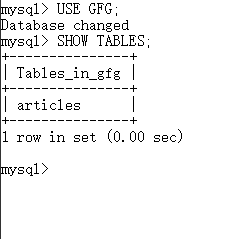Output:

```Loading required package: DBI
 "articles"```

## Create Tables in MySQL Using R

Tables in MySQL can be created using function dbWriteTable() in R. This function overwrites the table if table already exists.

Syntax: dbWriteTable(conn, name, value)

Parameter value:

• conn represents connection object
• name represents name of the table in MySQL
• value represents dataframe that has to be presented as MySQL table

Example:

## R

 `# Create connection object` `mysqlconn = ``dbConnect``(``MySQL``(), user = ``'root'``, ` `                      ``password = ``'welcome'``,` `                      ``dbname = ``'GFG'``,` `                      ``host = ``'localhost'``)`   `# Create new table mtcars` `dbWriteTable``(mysqlconn, ``"mtcars"``, ` `             ``mtcars[1:10, ],` `             ``overwrite = ``TRUE``)`

Output:

` TRUE`

Database table content: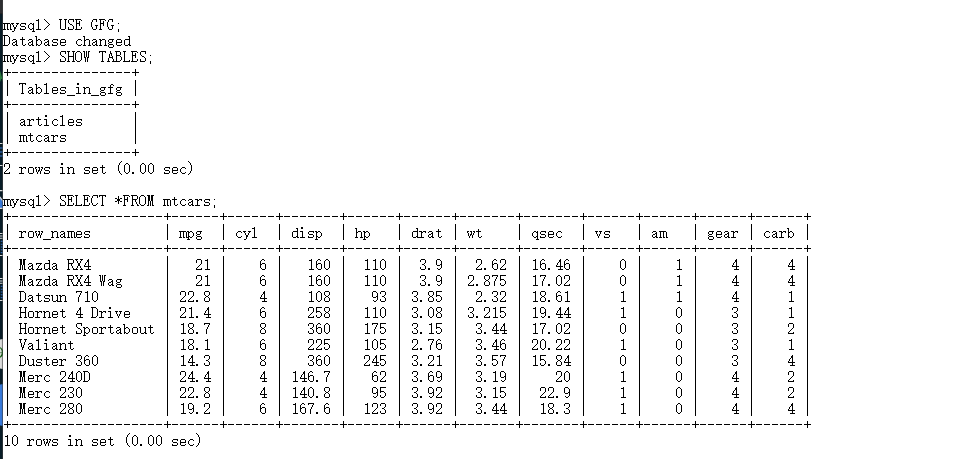## Drop Tables in MySQL Using R

To perform other operations than creating table, dbSendQuery() function is used to execute a query.

Syntax: dbSendQuery(conn, statement)

Parameter values:

• conn represents connection object
• statement represents query to be executed in MySQL

Example:

## R

 `# Create connection object` `mysqlconn = ``dbConnect``(``MySQL``(), user = ``'root'``,` `                      ``password = ``'welcome'``,` `                      ``dbname = ``'GFG'``, ` `                      ``host = ``'localhost'``)`   `# Drop table mtcars from database` `dbSendQuery``(mysqlconn, ``'DROP TABLE mtcars'``)`

Output:

`<MySQLResult:745348760, 3, 5>`

Database content: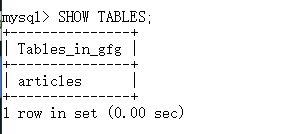## Insert into Table in MySQL Using R

Here we are going to insert a value into a table.

Example:

## R

 `# Create connection object` `mysqlconn = ``dbConnect``(``MySQL``(), user = ``'root'``,` `                      ``password = ``'welcome'``,` `                      ``dbname = ``'GFG'``, host = ``'localhost'``)`   `# Inserting into articles table` `dbSendQuery``(mysqlconn, "insert into ``articles``(sno, type) ` `values``(1, ``'R language'``)"` `)`

Output:

`<MySQLResult:745348760, 3, 6>`

Database content: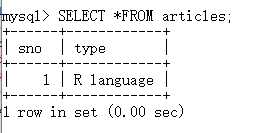## Updating a Table in MySQL Using R

Here we are going to update table in Mysql.

Example:

## R

 `# Create connection object` `mysqlconn = ``dbConnect``(``MySQL``(), user = ``'root'``, ` `                      ``password = ``'welcome'``,` `                      ``dbname = ``'GFG'``, host = ``'localhost'``)`   `# Update query` `dbSendQuery``(mysqlconn, "UPDATE articles SET sno = 10 \` `where type = ``'R language'``")`

Output:

`<MySQLResult:-1, 3, 7>`

Database content: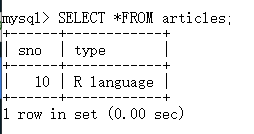#### Querying a Table in MySQL Using R

Here we are going to see how to use query in table.

Example:

## R

 `# Create connection object` `mysqlconn = ``dbConnect``(``MySQL``(), user = ``'root'``,` `                      ``password = ``'welcome'``,` `                      ``dbname = ``'GFG'``, host = ``'localhost'``)`   `# Select all rows from articles table` `res = ``dbSendQuery``(mysqlconn, ``"SELECT *FROM articles"``)`   `# Fetch first 3 rows in data frame` `df = ``fetch``(res, n = 3)` `print``(df)`

Output:

```  sno       type
1   1 Data Struc
2   2       Algo
3   3       Java```

Database content: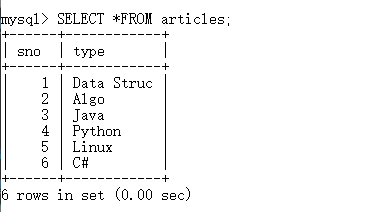My Personal Notes arrow_drop_up